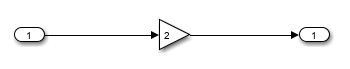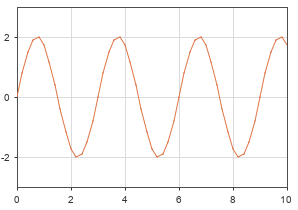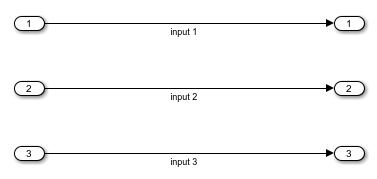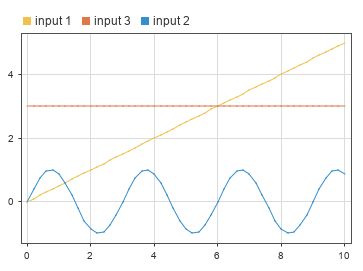# setExternalInput

Specify external input data for top-level input ports in simulation configured using `Simulink.SimulationInput` object

## Syntax

``simIn = setExternalInput(simIn,inData)``

## Description

example

````simIn = setExternalInput(simIn,inData)` adds the external input data `inData` to the simulation configuration in the `Simulink.SimulationInput` object `simIn`. When you run a simulation using a `SimulationInput` object that has external input data specified, the software selects the Input model configuration parameter and specifies the input data to load as `inData`.Use this function to specify external input data to load using top-level input ports in your model.```

## Examples

collapse all

Use the `setExternalInput` function to specify external data for the simulation configuration stored in a `Simulink.SimulationInput` object. Then, configure the Inport block to load the input data as a continuous signal using the `setBlockParameter` function. Finally, run the simulation using the `Simulink.SimulationInput` object.

Open the model `LoadInputData`. The model contains one Inport block that is connected to a Gain block with a Gain value of `2`. The output of the Gain block is connected to an Outport block.

```mdl = "LoadInputData"; open_system(mdl)```Create a `Simulink.SimulationInput` object for the model.

`simIn = Simulink.SimulationInput(mdl);`

Create input data to load using the Inport block. For this example, create data that represents a sine wave using an evenly spaced vector of time values. Then, use the `sin` function to create a signal value for each time point.

```sampleTime = 0.1; numSteps = 100; time = sampleTime*(0:numSteps); time = time'; data = sin(2*pi/3*time);```

You can specify external input data for top-level input ports using several formats. For this example, use the `timetable` format. To create a `timetable`, the time data must be a `duration` vector. Use the `seconds` function to create a `duration` vector with units of seconds. Then, create the `timetable`.

```secs = seconds(time); inData = timetable(secs,data);```

Use the `setExternalInput` function to add the external input data `inData` to the simulation configuration in the `Simulink.SimulationInput` object `simIn`.

`simIn = setExternalInput(simIn,inData);`

You can also configure other aspects of the simulation using the `Simulink.SimulationInput` object, such as block parameter values, model configuration parameter values, and variable values.

Suppose you want to configure the Inport block to load the input data as a continuous signal. Use the `setBlockParameter` function to enable the Interpolation parameter and specify the Sample time parameter for the block as continuous (`0`).

```inBlk = mdl + "/Inport"; simIn = setBlockParameter(simIn,inBlk,"Interpolate","on"); simIn = setBlockParameter(simIn,inBlk,"SampleTime","0");```

Simulate the model using the configuration on the `SimulationInput` object.

`out = sim(simIn);`When your model contains several top-level input ports, you can specify the input data to load as a `Simulink.SimulationData.Dataset` object that contains an element with the data for each input port. The first element in the object contains the data for the input port with a port number of `1`, the second element contains the data for the input port with a port number of `2`, and so on.

When you specify the data to load using the variable that contains the data, the data in the variable you specify is added to the `Simulink.SimulationInput` object so the data is available for simulations run using the object.

This example shows how to load data for three top-level input ports into a simulation configured using a `SimulationInput` object. The input data for each input port uses a different format to illustrate several available formats.

Open the model `InportLoading`. The model contains three Inport blocks that are connected directly to three Outport blocks.

```mdl = "InportLoading"; open_system(mdl)```Use the `createInputDataset` function to create a `Dataset` object that contains an element for each input port in the model.

`inDS = createInputDataset(mdl);`

By default, the `Dataset` object created by the `createInputDataset` function contains placeholder `timeseries` objects for each element. Create input data for each input port to replace the placeholder data.

For the first input port, create a `timeseries` object with data that represents a line with a slope of `0.5`. For the time data, create an evenly spaced time vector using a sample time, or spacing, of `0.5`. To create the signal values, multiply the time vector by `0.5`.

```sampleTime = 0.5; numSteps = 20; time = sampleTime*(0:numSteps)'; data = 0.5*time; lineTS = timeseries(data,time);```

For the second input port, create a `timetable` with data that represents a sine wave.

1. For the sine wave, create an evenly spaced time vector using a sample time of `0.1`.

2. To create the signal values, use the `sin` function.

3. To create a `timetable`, the time input must be a `duration` vector. Use the `seconds` function to convert the time values into a `duration` vector with units of seconds.

4. Create the `timetable`.

```sampleTime = 0.1; numSteps = 100; time = sampleTime*(0:numSteps)'; sinVals = sin(2*pi/3*time); secs = seconds(time); sinTT = timetable(secs,sinVals);```

For the third input port, create a structure that contains data for a constant with a value of `3`. For input data loading, the structure format has the fields `time` and `signals`. The `signals` field is an array of structures that each contain the data for a signal in the field `values`. For this example, the `signals` array has only one element because the structure specifies input data for one input port.

```constStruct.time = [0 10]'; constStruct.signals.values = [3 3]';```

Replace the placeholder data with the data created for each input port.

```inDS{1} = lineTS; inDS{2} = sinTT; inDS{3} = constStruct;```

Create a `Simulink.SimulationInput` object to configure a simulation of the model.

`simIn = Simulink.SimulationInput(mdl);`

Use the `setExternalInput` function to specify the input data to load for all three input ports using the `Dataset` object. The function adds the variable `inDs` to the `SimulationInput` object.

`simIn = setExternalInput(simIn,inDS);`

Simulate the model using the `SimulationInput` object.

`out = sim(simIn);`

The simulation runs, loading the input data as specified on the `SimulationInput` object.## Input Arguments

collapse all

Simulation inputs and configuration, specified as a `Simulink.SimulationInput` object.

External input data for top-level input ports, specified using a format supported by the Input parameter.

When your model contains multiple top-level input ports, specify the data for all input ports using a single variable that contains the data for all top-level input ports. When you specify the external input data to load as a variable, the data in the variable is added to the `SimulationInput` object and is available for any simulation you run using the object, including simulations on local or remote workers.

You can use several formats to store the input data for all top-level input ports in a single variable, including:

• `Simulink.SimulationData.Dataset` — Each element in the `Dataset` object specifies the input data for a top-level input port.

• `Simulink.SimulationData.DatasetRef` — Stream data from a `Dataset` object saved in a MAT file.

• Structure with or without time — Each structure in the `signals` field of the input structure specifies the input data for a top-level input port.

• Array — The first column specifies time data for all signals. Each subsequent column specifies the scalar signal values for a top-level input port.

The software maps the signal data in the variable to each top-level input port based on the order of the signal data within the variable and the port number. For example, the first element in a `Dataset` object maps to the top-level input port with a port number of `1`.

You can format the data for each top-level input port to load using several formats, including:

• `timeseries`

• `timetable`

Each timetable object must contain one column with data for a single signal.

• Structure with or without time — The structure defines input data for only one input port in this case.

• Array — The array defines input data for only one input port in this case.

#### Comma-Separated List

Specifying input data for a `Simulink.SimulationInput` object as a comma-separated list is generally not recommended.

When you specify the input data as a comma-separated list, the `setExternalInput` function does not add the variables that contain the data to the `SimulationInput` object. You must ensure that the input data is available for the simulations you run using the `SimulationInput` object by managing the data in the workspace or by adding the input data to the `SimulationInput` object yourself using the `setVariable` function.

## Output Arguments

collapse all

Simulation configuration with external inputs added, returned as a `Simulink.SimulationInput` object.

## Tips

• To specify input data to load for a block that has parameter to specify the data to load from the workspace, specify the block parameter value as a variable. Then, use the `setVariable` function to specify the value for the variable in the simulation configuration represented by the `SimulationInput` object.

For example, to specify the data to load for a From Workspace block, specify the Data parameter for the From Workspace block as a variable. Then, use the `setVariable` function to specify the value of the variable.

• To load input data from a file, ensure that the simulation can access the file that contains the input data. When you run simulations using the `parsim` and `batchsim` functions, you can send files to the workers using the `AttachedFiles` name-value argument.

## Version History

Introduced in R2017a